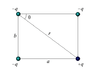# Superposition of 3 forces on a point charge

• jenzao

## Homework Statement

Four charges are placed on the corners of a rectangle. What is the resultant force on the positive charge (a = 1.5 m, b = 0.5 m, q = 1.1 × 10-9C)?
(see attached drawing)

## Homework Equations

Im having problems summing the forces using superposition

## The Attempt at a Solution

F = k(qq) / r^2

F1 = 4e-9 (r=1.5m)
F2 = 4e-8 (r=0.5m)
F3 = 4e-9 (r=1.58m)

then when i try to superimpose i get probs..
i put tail of F1 vector at origin, then put tail of F2 vector ontop tip of F1.
I do the same for F3.
then i draw line from tail of F1 to tip of F3.
this is equal to 2*F3.
= wrong

#### Attachments

•showme.gif
2.8 KB · Views: 445

I'll suggest figuring out the horizontal and vertical component of each vector (using sine and cosine), and find the resultant by adding up components.

F1 = 4e-9 (r=1.5m)
F2 = 4e-8 (r=0.5m)
F3 = 4e-9 (r=1.58m)

What is the direction of each of these vectors?

Also, you should check your arithmetic and keep more digits until you reach the final answers. For F1 I get 4.84e-9 which is a big difference!

Always keep more digits during the intermediate steps, until you reach your final answer, and only then round it off to the proper number of digits.

Last edited: